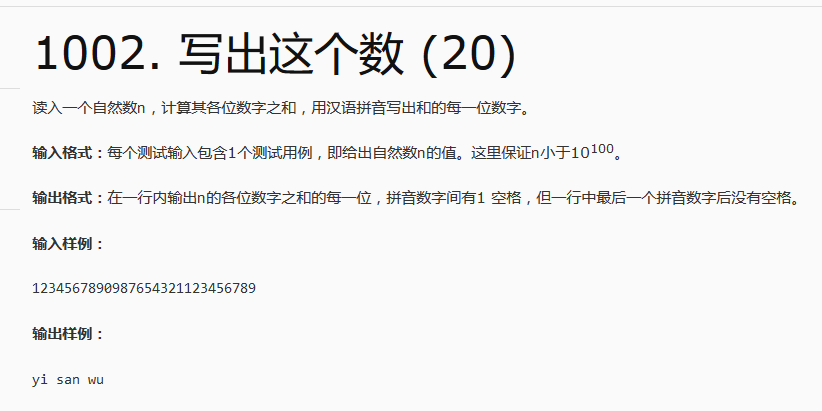# PAT乙级1002. 写出这个数 C++#include <iostream>
#include <string>
using namespace std;
void static readNumber(char char_number);
int main()
{
string input;//输入的数字
cin>>input;
unsigned int sum=0;//和
//求和
for(unsigned int i=0;i<input.length();i++)
{
sum+=(unsigned int)input[i]-48;
}

//装入倒序的结果字符串
string number_sum="";
while(1)
{
number_sum+=(char)(sum%10+48);
sum/=10;
if(sum/10==0)//当这个运算进行到还剩最后一位的时候，也有可能是只有一位，并且还是零
{
if(sum%10!=0)//从这里，我们考虑是零的边界情况，只有这一位不是零的时候才装入
{
number_sum+=(char)(sum%10+48);
}
break;
}
}
//变为正序字符串，装入结果集
string result="";
for(int i=number_sum.length()-1;i>=0;i--)
{
result+=number_sum[i];
}
//输出结果
for(int i=0;i<result.length();i++)
{
readNumber(result[i]);
if(i!=result.length()-1)//当且仅当遍历输出的数字不是最后一个的时候才打印空格
{
cout<<" ";
}else
{

}

}
system("pause");
return 0;
}

void static readNumber(char char_number)
{
switch((int)char_number-48)
{
case 0:
cout<<"ling";
break;
case 1:
cout<<"yi";
break;
case 2:
cout<<"er";
break;
case 3:
cout<<"san";
break;
case 4:
cout<<"si";
break;
case 5:
cout<<"wu";
break;
case 6:
cout<<"liu";
break;
case 7:
cout<<"qi";
break;
case 8:
cout<<"ba";
break;
case 9:
cout<<"jiu";
break;
}
}